#### Probabilistic forward projections

The probabilistic forward projection can be considered as a Markov process because the decision'' part is removed once the actions are given. Suppose that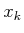is given and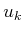is applied. What is the probability distribution over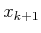? This was already specified in (10.6) and is the one-stage forward projection. Now consider the two-stage probabilistic forward projection,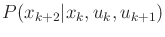. This can be computed by marginalization as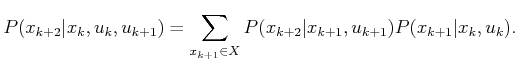(10.13)

Computing further forward projections requires nested summations, which marginalize all of the intermediate states. For example, the three-stage forward projection is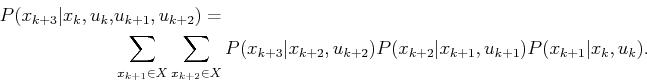(10.14)

A convenient expression of the probabilistic forward projections can be obtained by borrowing nice algebraic properties from linear algebra. For each action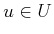, let its state transition matrix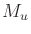be an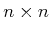matrix, for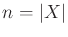, of probabilities. The matrix is defined as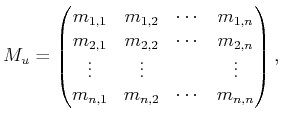(10.15)

in which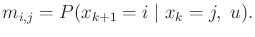(10.16)

For each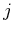, theth column ofmust sum to one and can be interpreted as the probability distribution overthat is obtained ifis applied from state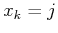.

Let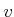denote an-dimensional column vector that represents any probability distribution over. The product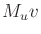yields a column vector that represents the probability distribution overthat is obtained after starting withand applying. The matrix multiplication performsinner products, each of which is a marginalization as shown in (10.13). The forward projection at any stage,, can now be expressed using a product of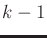state transition matrices. Suppose that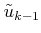is fixed. Let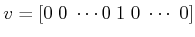, which indicates that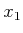is known (with probability one). The forward projection can be computed as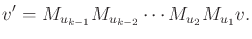(10.17)

Theth element of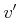is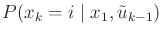.

Example 10..4 (Probabilistic Forward Projections)   Once again, use the first model from Example 10.1; however, now assign probability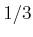to each nature action. Assume that, initially,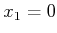, and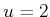is applied in every stage. The one-stage forward projection yields probabilities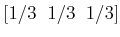(10.18)

over the sequence of states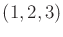. The two-stage forward projection yields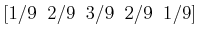(10.19)

over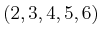.Steven M LaValle 2020-08-14